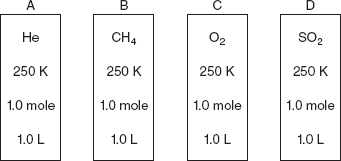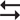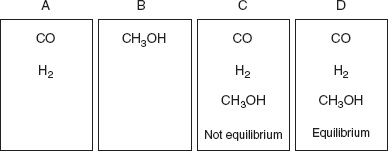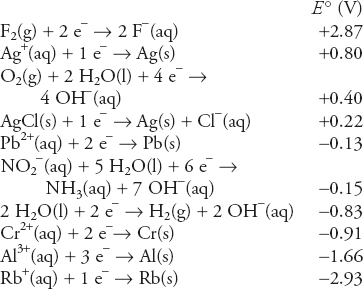# AP Chemistry Practice Test 39

### Test Information14 questions21 minutes

`Questions 1-3 refer to the following information.Use the information on the containers in the following diagram to answer questions.Approximate molar masses:He = 4 g mol-1, CH4 = 16 g mol-1,O2 = 32 g mol-1, SO2 = 64 g mol-1`

1. Under the conditions indicated, in which of the gas sample is the average velocity of the molecules half that of methane, CH4?

2. Which of the four gases will probably show the least deviation from ideal behavior?

3. If one of the containers sprang a small leak, which of the following would change?

4. The specific rate constant, k, for a certain first-order reaction is 86 h-1. What mass of a 0.0500 g sample of starting material will remain after 58 s?

`Questions 5-9 refer to the following information.Use the information on the containers in the following diagram to answer questions concerning the following equilibrium:CO(g) + 2 H2(g)CH3OH(g)`

5. Container A initially contains 0.60 mole of H2 and 0.60 mole of CO and is allowed to come to equilibrium. At equilibrium, there are 0.40 mole of CO in the flask. What is the value of Kc, the equilibrium constant, for the reaction?

6. A 1.00-mole sample of CH3OH is placed in container B and the system is allowed to go to equilibrium. What can be said about the relative rates of reaction with respect to the various components?

7. The mixture in container D is in equilibrium. Which of the following is true?

8. The mixture in container A goes to equilibrium. If the initial moles of H2(g) is twice the initial moles of CO(g), which of the following is true?

9. As the mixture in container B approaches equilibrium, the partial pressure of CH3OH gas decreases by 1.5 atm. What is the net change in the total pressure of the system?

`Questions 10-14 refer to the following information.Use the information on standard reduction potentials in the following table to answer questions.`

10. A student constructs an electrolysis cell with two inert electrodes in an aqueous solution that is 1.0 M in rubidium nitrite, RbNO2, and 1.0 M in rubidium hydroxide, RbOH. As the cell operates, an odorless gas evolves from one electrode and a gas with a distinctive odor evolves from the other electrode. Choose the correct statement from the following list.

11. There is a galvanic cell involving a lead, Pb, electrode in a 1.0 M lead(II) nitrate, Pb(NO3)2, solution and a chromium, Cr, electrode in a 1.0 M chromium(II) sulfate, CrSO4, solution. What is the cell potential?

12. A student attempted to prepare an electrolysis cell to produce aluminum metal (Al) from an aqueous solution of aluminum chloride, AlCl3, using a 6.0 V battery. The cathode compartment of the electrolysis contained 1.0 M aluminum chloride. The student was unsuccessful. Why was the student unable to produce aluminum metal?

13. Which of the substances in the table would be capable of reducing the aluminum ions in solid aluminum fluoride, AlF3, to aluminum metal? Assume the cell potentials in the table also apply to the solid state.

14. A student constructs a galvanic cell that has a chromium, Cr, electrode in a compartment containing a 1.0 M chromium(II) nitrate, Cr(NO3)2, solution and a silver, Ag, electrode in a compartment containing 1.0 M silver nitrate, AgNO3, solution. A salt bridge containing a 1.0 M potassium chloride, KCl, solution connects the two compartments. When the student measures the cell potential, the value is far from the ideal predicted value. What is the cause of this discrepancy?